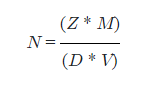Request a Tool

The Avogadro’s number is the unit in one mole of any substance

0

Formula• Z = Number of atoms within the crystal unit cell
• M = Average atomic mass
• D = Density
• V = Volume

Defination / Uses

Number 6.022 × 10^23 indicating the number of (atoms or molecules) in a mole of any substance is called Avogadro's.

Avogadro's number are used in chemistry when you need to work with very long numbers. i.e 3.35 x 10^19 H2O molecules in a 1mg snowflake.

The units of Avogadro's number is mol-1.